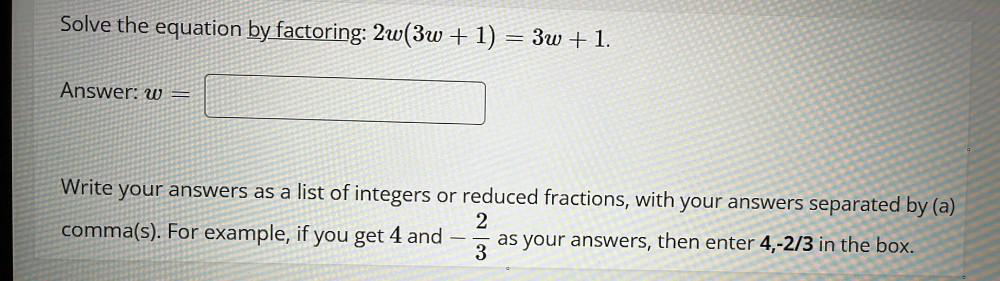Question:

# Solve the equation by factoring: 2w(3w + 1) = 3w + 1. w= Write your answers as a list of integers or reduced fractions, with your answers separated by (a) comma(s). For example, if you get 4 and -2/3Solve the equation by factoring: 2w(3w + 1) = 3w + 1. w= Write your answers as a list of integers or reduced fractions, with your answers separated by (a) comma(s). For example, if you get 4 and -2/3 and as your answers, then enter 4,-2/3 in the box.